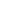# Calculating Time-dependent Routemetrics

Timedependent routeA route corresponds to a path of a vehicle through the underlying transport network. The main attributes of a route are the distance and the time that the vehicle travels along the path. metrics are proposed comparative figures which can be used for better explanation of the results calculated by taking into account timedependent data. Hence the figures could increase the acceptance by the user of a timedependent route calculation

### Benefits

• Realtime route calculation
• Better acceptance of calculated routes through comparative figures

### Prerequisites

Check if the following prerequisites are fulfilled before you start with the use case.

• Installed and licensed PTV xRoute service.

• Installed map includes Luxembourg by HERE with the Feature Layer themes PTV_SpeedPatterns and PTV_TrafficIncidents.

### Concepts

Then following time dependent route metrics can be calculated:

• Let `Ro` be the optimistic route; i.e. best route without taking into account the current traffic situation
• Let `to(Ro)` be the optimistic traveltime on optimistic route; i.e. the needed traveltime to drive on the optimistic route `Ro` not taking into account the current traffic situation
• Let `te(Ro)` be the exact traveltime on optimistic route; i.e. the needed traveltime to drive on the optimistic route `Ro` taking into account current traffic situation
• Let `Re` be the exact route; i.e. the best route taking into account the current traffic situation. In general this results in a detour compared with the optimistic route `Ro`
• Let `te(Re)` be the exact traveltime on exact route; i.e. the needed traveltime to drive on the exact route `Re` taking into account current traffic situation
• Let `to(Re)` be the optimistic traveltime on exact route; i.e. the needed traveltime to drive on the exact route `Re` not taking into account current traffic situation
• Let `detourBenefit=te(Ro)-te(Re)` be the benefit of driving a detour around congested roads in contrast to driving over them. The detour is not necessarily longer than the original route, but the crucial point is that the detour is different from the original route.
• Let `delayDueToTrafficSituation=te(Ro)-to(Ro)` be the delay caused by the congestion when driving through it in contrast to the route without any congestion.
• Let `delayDueToTrafficSituationWithDetour=te(Re)-to(Ro)` be the delay caused by the congestion when driving around it in contrast to the route without any congestion.

To calculate all these metrics, the following technical concepts are used:

• Time consideration scenarios are used to swicth between optimistic and exact calculation.
• Encoded path is used to recalculate the traveltime on a precomputed route.

### Programming Guide

// Data var referenceTime = "2017-01-09T07:00:00+01:00"; // Map var map = new L.Map('map', { center: [49.6385, 5.9847], zoom: 13 }); // Add tile layer to map new L.tileLayer.xserver(xServerUrl + '/services/rest/XMap/tile/{z}/{x}/{y}' + '?layers=background,transport,labels,PTV_SpeedPatterns,PTV_TrafficIncidents' + '&timeConsideration=SNAPSHOT&referenceTime=' + encodeURIComponent(referenceTime) + '&showOnlyRelevantByTime=false' + '&contentType=JSON', { pane: "overlayPane", maxZoom: 20 }).addTo(map); var A = { "\$type": "OffRoadWaypoint", "location": { "offRoadCoordinate": { "x": 5.9556, "y": 49.6468 } } }; var B = { "\$type": "OffRoadWaypoint", "location": { "offRoadCoordinate": { "x": 6.0141, "y": 49.6355 } } }; var outputString = ''; var outputencodedPath = ''; var to_ro = 0; var td_ro = 0; var td_rd = 0; var to_rd = 0; function calculateSpecificRoute(tct, waypoints, routeOptions) { xroute.calculateRoute({ "storedProfile":"car", "requestProfile": { "featureLayerProfile": { "themes": [{ "id": "PTV_SpeedPatterns", "enabled": true },{ "id": "PTV_TrafficIncidents", "enabled": true }] } }, "waypoints": waypoints, "routeOptions": routeOptions, "resultFields": { "polyline": true, "encodedPath": true }, "geometryOptions": { "responseGeometryTypes": ["GEOJSON"] } }, function(route, exception) { outputencodedPath = route.encodedPath; var geoJson = route.polyline.geoJSON; if(tct == "ExactTimeConsiderationAtStart") { displayGeoJson(geoJson, '#ffa225'); } else { displayGeoJson(geoJson, '#2882C8'); } if(to_ro == 0 && td_ro == 0 && td_rd == 0 && to_rd == 0){ to_ro = route.travelTime; var EncodedPath = { "\$type": "PathWaypoint", "encodedPath": outputencodedPath }; var waypoints = [EncodedPath]; var routeOpt = { "timeConsideration": { "\$type": "ExactTimeConsiderationAtStart", "referenceTime": referenceTime } }; calculateSpecificRoute("ExactTimeConsiderationAtStart",waypoints, routeOpt); } else if(to_ro != 0 && td_ro == 0 && td_rd == 0 && to_rd == 0){ td_ro = route.travelTime; var waypoints = [A, B]; var routeOpt = { "timeConsideration": { "\$type": "ExactTimeConsiderationAtStart", "referenceTime": referenceTime } }; calculateSpecificRoute("OptimisticTimeConsideration", waypoints, routeOpt); } else if(to_ro != 0 && td_ro != 0 && td_rd == 0 && to_rd == 0){ td_rd = route.travelTime; var EncodedPath = { "\$type": "PathWaypoint", "encodedPath": outputencodedPath }; var waypoints = [EncodedPath]; var routeOpt = { "timeConsideration": { "\$type": "OptimisticTimeConsideration" } }; calculateSpecificRoute("OptimisticTimeConsideration", waypoints, routeOpt); } else if(to_ro != 0 && td_ro != 0 && td_rd != 0 && to_rd == 0){ to_rd = route.travelTime; var detourBenefit = Number(Math.abs(td_ro - td_rd)).toFixed(2) ; var delayDueToTrafficSituation = Number(td_ro-to_ro).toFixed(2); var delayDueToTrafficSituationWithDetour = Number(td_rd-to_ro).toFixed(2); outputString += " detourBenefit: " + detourBenefit; outputString += "s delayDueToTrafficSituation: " + delayDueToTrafficSituation; outputString += "s delayDueToTrafficSituationWithDetour: " + delayDueToTrafficSituationWithDetour; print(outputString); } }); } function displayGeoJson(geoJson,color) { var jsonObject = JSON.parse(geoJson); var geoJsonLayer = new L.GeoJSON(jsonObject, { style: { color: color, weight: 8 } }).addTo(map); }; var waypoints = [A, B]; var routeOpt = { "timeConsideration": { "\$type": "OptimisticTimeConsideration" } }; calculateSpecificRoute("OptimisticTimeConsideration", waypoints, routeOpt);